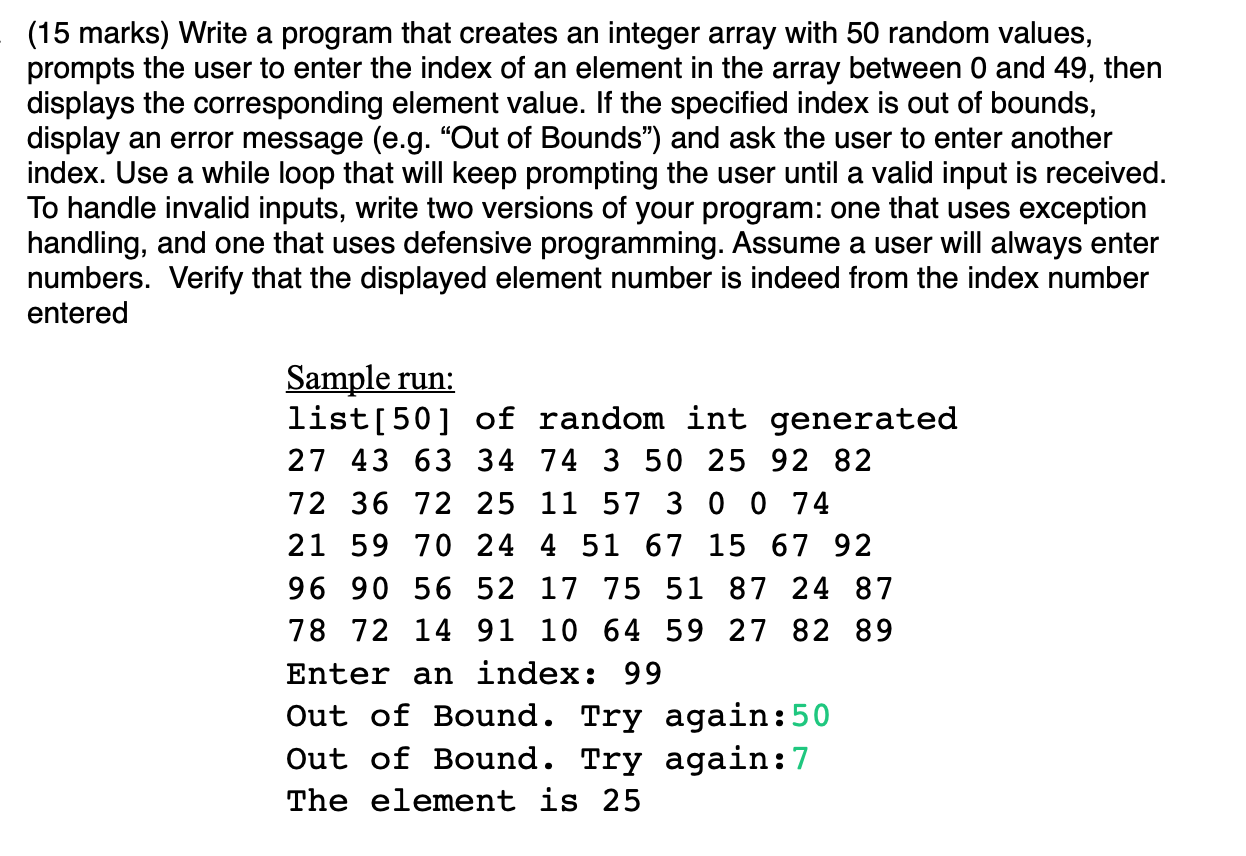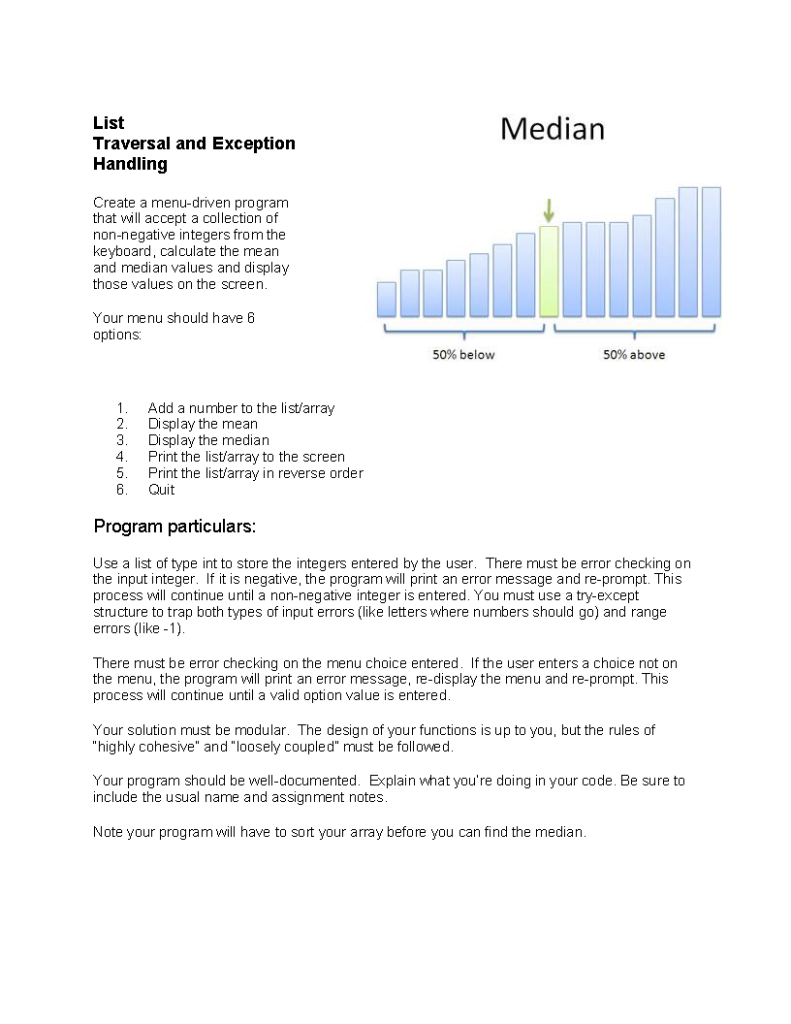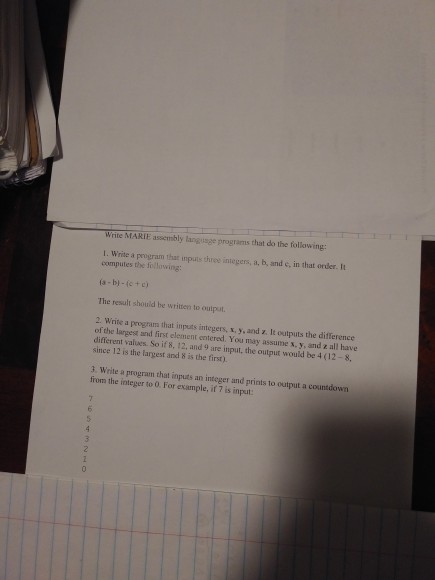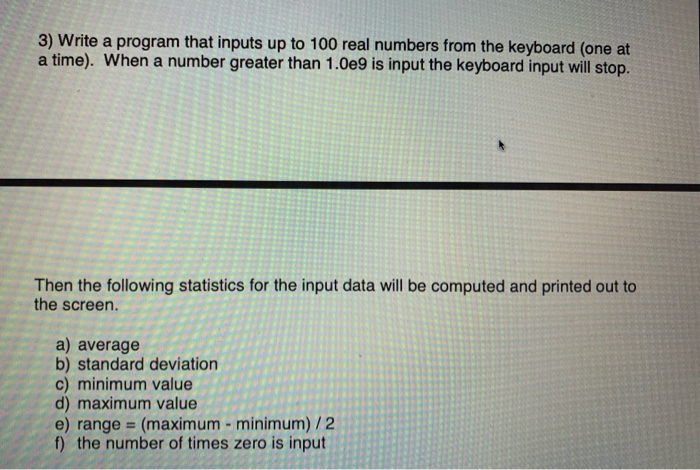Question

# Write a Java program that inputs a list of integer values in the range of −...

Write a Java program that inputs a list of integer values in the range of − 1 to 100 from the keyboard and computes the sum of the squares of the input values. This program must use exception handling to ensure that the input values are in range and are legal integers, to handle the error of the sum of the squares becoming larger than a standard Integer variable can store, and to detect end-of-file and use it to cause the output of the result. In the case of overflow of the sum, an error message must be printed, and the program terminated.

// JAVA Code as shown below //

import java.util.ArrayList;

import java.util.Scanner;

import java.lang.Exception;

public class SumofSquares

{

//main function start

public static void main(String[] args)

{

//creating arraylist named numbers to store input values

ArrayList<Integer> numbers = new ArrayList<Integer>();

Scanner in = new Scanner(System.in);

System.out.println("Please enter a list of numbers between -1 to 100 ");

try

{

//checks the entered number between 100 to -1

while ((in.hasNextInt()<=100)&& (in.hasNextInt()>=-1))

{

int input = in.nextInt();

}

}//try

catch(InputMismatchException exception)

{

System.out.println("This is not an integer");

}

catch(Exception e){

System.out.println("number not in the range");

}

if (numbers.size() == 0)

{

}

else

{

int total = 0;

for (int element : numbers)

{

try{

total = total + element*element;

}//try

catch(Exception ex){

System.out.println("sum of squares of given list is out of bound");

}//catch

}

System.out.println("The SumOfSquares is: " + total);

}

}

}

#### Earn Coins

Coins can be redeemed for fabulous gifts.

Similar Homework Help Questions
• ### using C++ Write a program that: a) Inputs an integer n from the keyboard where n<=100....

using C++ Write a program that: a) Inputs an integer n from the keyboard where n<=100. If n is out of range then print out an error message and ask for another input. This process repeats until a valid value for n is obtained. b) Inputs two 1D arrays of doubles A and B (of size n) from the keyboard. c) Inputs an integer k (from 1 to 3) from the keyboard. d) If k = 1 then it calculates...

• ### Part I Create a Java program that inputs a grade from the user. The grade input...

Part I Create a Java program that inputs a grade from the user. The grade input from the user will be an integer. Once the input is stored, use an if-else-if block of code to determine the letter grade of the inputted integer grade. Do not use a bunch of if statements by themselves to solve this problem. You will print an error message for inputs greater than 100 and for inputs less than 0. Both errors must be handled...

• ### JAVA: (15 marks) Write a program that creates an integer array with 50 random values, prompts...JAVA: (15 marks) Write a program that creates an integer array with 50 random values, prompts the user to enter the index of an element in the array between 0 and 49, then displays the corresponding element value. If the specified index is out of bounds, display an error message (e.g. "Out of Bounds") and ask the user to enter another index. Use a while loop that will keep prompting the user until a valid input is received. To handle...

• ### Java programming only Create a Java program that inputs a grade from the user. The grade input from the user will be an...

Java programming only Create a Java program that inputs a grade from the user. The grade input from the user will be an integer. Once the input is stored, use an if-else-if block of code to determine the letter grade of the inputted integer grade. Do not use a bunch of if statements by themselves to solve this problem. You will print an error message for inputs greater than 100 and for inputs less than 0. Both errors must be...

• ### Python 3.7 to be used. Just a simple design a program that depends on its own. You should also not need to import anythi...Python 3.7 to be used. Just a simple design a program that depends on its own. You should also not need to import anything. No code outside of a function! Median List Traversal and Exception Handling Create a menu-driven program that will accept a collection of non-negative integers from the keyboard, calculate the mean and median values and display those values on the screen. Your menu should have 6 options 50% below 50% above Add a number to the list/array...

• ### Write MARIE assembly language programs that do the following: I. Write a program that inputs thre...Write MARIE assembly language programs that do the following: I. Write a program that inputs three integers, a, b, and c, in that order. It computes the following ia-bi-fc+ c The result should be written to output 2. Write a program that inputs integers, s. y, and z. It outputs the difference of the langest and first element entered. You may assume x. y, and z all have different values. So if 8, 12, and 9 are input, the output...

• ### Write a program that reads in n2 integer values from the user and tests whether they form a...

Problem 1:Magic Squares:An n x n array, that is filled with integers 1, 2, 3, …,n2 is a magic square if the sum of the elements in each row, in each column, and in the two diagonals is thesame value and each value in the array is unique.16 3 2 135 10 11 89 6 7 124 15 14 1Write a program that reads in n2 integer values from the user and tests whether they form a magic square when...

• ### For Java Write a program that reads a sequence of integer inputs all on one line...

For Java Write a program that reads a sequence of integer inputs all on one line and prints: 1.)The smallest and largest of the inputs 2.)The number of odd and even inputs 3.)Cumulative totals. If the input is 1 7 2 9, the program prints 1 8 10 19. 4.)All adjacent duplicates. If the input is 1 3 3 4 5 5 6 6 6 2, the output is 3 5 6.

• ### Write a program that inputs up to 100 real numbers from the keyboard (one at a...Write a program that inputs up to 100 real numbers from the keyboard (one at a time). When a number greater than 1.0e9 is input the keyboard input will stop. Then the following statistic for the input data will be computed and printed out to the screen. a) average b) standard deviation c) minimum value d) maximum value e) range = (maximum-minimum)/2 f) the number of times zero is input **Note: this is a program in C++** 3) Write a...

• ### Part A Write a Java program that reads an integer n from the keyboard and loops...

Part A Write a Java program that reads an integer n from the keyboard and loops until −13 ≤ n ≤ 13 is successfully entered. A do-while loop is advised. Part B Write a Java program that reads an integer n from the keyboard and prints the corresponding value n!. [This is n factorial]. You must verify that the input integer satisfies the constraint 0 ≤ n ≤ 13; keep looping until the constraint is satisfied.   Will give thumbs up...